## 4.1.4 The Drift-Diffusion Transport Model

The drift-diffusion transport model is the simplest macroscopic transport model based on the solution of the semi-classical Boltzmann's transport equation. Together with higher-order macroscopic transport models it is only valid in device operation modes, where quantum effects are negligible. In order to account for such effects several quantum mechanical models were developed. Those, however are beyond the scope of this work and a detailed discussion can be found e.g. in .

There are two methods to derive the DD transport model from the BTE: by the method of the moments  and from basic principles of irreversible thermodynamics . In the former the BTE is multiplied by a set of weight functions and integrated over the momentum space. The DD transport model takes only the first two moments into account. However, specific to the moments method, each equation of a given moment contains also the next higher moment. Thus, the equation system is under-defined. In order to address this problem (the so called closure problem), the carriers are assumed to be in thermal equilibrium with the lattice (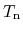=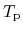=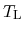). This yields: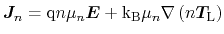(4.15)

for electrons, where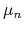is the electron mobility and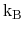is the Boltzmann constant. From now on the equivalent equations for holes are omitted as they can be easily derived from those for electrons.

The DD transport model was the de-facto standard model for semiconductor device simulations for many years due to its excellent convergence behavior and relatively low computational cost. However, its major drawback is that it cannot account for non-local effects such as e.g. velocity overshoot and real-space transfer.

S. Vitanov: Simulation of High Electron Mobility Transistors